1800-1023-196

+91-120-4616500

# Ion-Electron Method (Half Reaction Method)﻿

## Ion-Electron Method (Half Reaction Method)

Jette and LaMev developed the method for balancing redox-reactions by ion electron method in 1927. It involves the following steps

(i) Write down the redox reaction in ionic form.

(ii) Split the redox reaction into two half reactions, one for oxidation and other for reduction.

(iii) Balance each half reaction for the number of atoms of each element. For this purpose,

(a) Balance the atoms other than H and O for each half reaction using simple multiples.

(b) Add water molecules to the side deficient in oxygen and H+ to the side deficient in hydrogen. This is done in acidic or neutral solutions.

(c) In alkaline solution, for each excess of oxygen, add one water molecule to the same side and 2OH ions to the other side. If hydrogen is still unbalanced, add one OH ion for each excess hydrogen on the same side and one water molecule to the other side.

(iv) Add electrons to the side deficient in electrons as to equalise the charge on both sides.

(v) Multiply one or both the half reactions by a suitable number so that number of electrons become equal in both the equations.

(vi) Add the two balanced half reactions and cancel any term common to both sides.

The following example illustrate the above rules

Step: I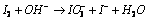(Ionic equation)

Step: II Splitting into two half reactions,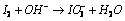;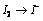(Oxidation half reaction) (Reduction half reaction)

Step: III Adding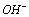ions,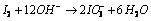Step: IV Adding electrons to the sides deficient in electrons, (Si)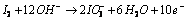;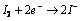Step: V Balancing electrons in both the half reactions.;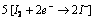Step: VI Adding both the half reactions.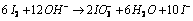;

Dividing by 2,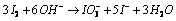## NEET & AIIMS Exam Sample Papers

 AIIMS SAMPLE PAPERS View More NEET SAMPLE PAPERS View More